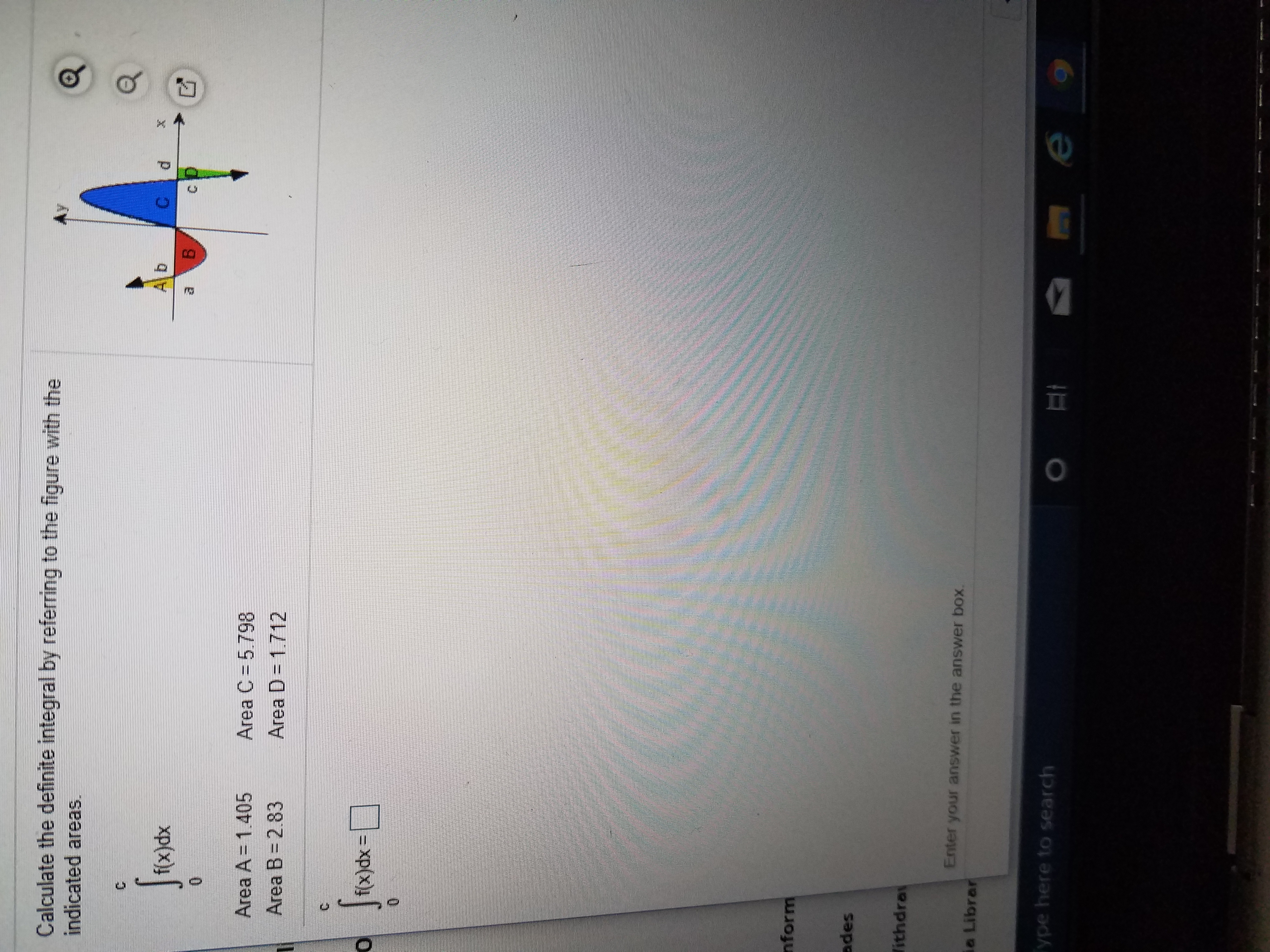# Calculate the definite integral by referring to the figure with theindicated areas.f(x)dxB.Area C = 5.798Area D = 1.712Area A= 1.405Area B= 2.83f(x)dx%3DnformadesithdraEnter your answer in the answer box.ia LibrarType here to search

Question
34 viewshelp_outlineImage TranscriptioncloseCalculate the definite integral by referring to the figure with the indicated areas. f(x)dx B. Area C = 5.798 Area D = 1.712 Area A= 1.405 Area B= 2.83 f(x)dx%3D nform ades ithdra Enter your answer in the answer box. ia Librar Type here to search fullscreen
check_circle

Step 1

Given:

Area A=1.405

Area B=2.83

Area C=5....

### Want to see the full answer?

See Solution

#### Want to see this answer and more?

Solutions are written by subject experts who are available 24/7. Questions are typically answered within 1 hour.*

See Solution
*Response times may vary by subject and question.
Tagged in

### Other